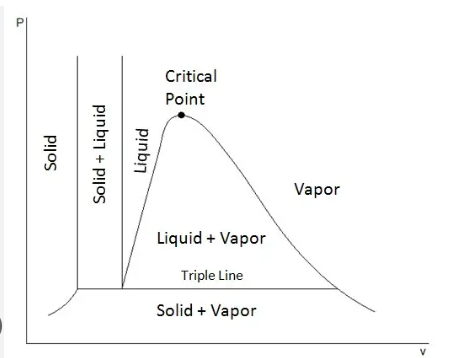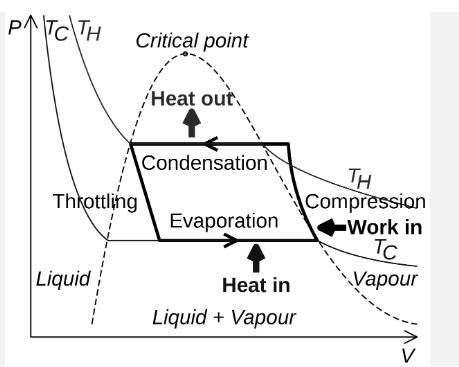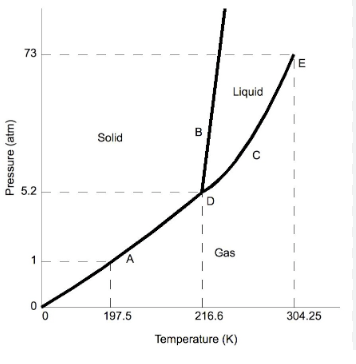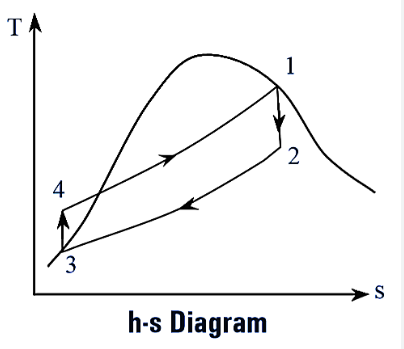Select Page

# Pure Substance

Pure Substance

Contents

Recall the P-V diagram for Phase change 2

Recall the concept of Latent Heat and Sensible Heat 3

Derive the expression for various regions in the T-S diagram 4

Recall the concept of the following: i. Quality Dryness Fraction ii. Critical Point 6

Derive an expression for Wet region 7

Recall the concept of Triple Point on P-T diagram 8

Recall the concept of H-S diagram 10

# Recall the concept of Phase change phenomena

The term phase change phenomena refers to the changes that occur in matter as it transitions between different states of matter, such as solid, liquid, and gas. In thermodynamics, phase change is important because it can involve the transfer of heat and energy, which can impact the performance of various systems and devices. For example, when a solid material is heated, it can change phase to become a liquid, releasing heat energy in the process. Similarly, when a liquid is cooled, it can change phase to become a solid, absorbing heat energy. The phase change process can be described using the concept of latent heat, which is the amount of heat energy that is required to change the phase of a substance without changing its temperature. Understanding phase change phenomena is essential for understanding the behavior of matter in different thermodynamic systems, and for designing and optimising various systems and devices in fields such as energy, power generation, refrigeration, and air conditioning.

# Recall the P-V diagram for Phase change

The P-V (Pressure-Volume) diagram is a graphical representation of the relationship between pressure and volume of a substance during a phase change process. In thermodynamics, phase changes occur when a substance changes from one state of matter to another, such as solid to liquid, liquid to gas, or gas to solid. The P-V diagram shows how the pressure and volume of a substance change during these phase changes, which is a useful tool for understanding the behavior of matter during these processes.

In the P-V diagram, the horizontal axis represents the volume of a substance and the vertical axis represents the pressure. During a phase change process, the substance follows a particular path on the diagram, which is determined by the specific conditions of the process, such as the initial and final temperature and pressure of the substance.For example, during a solid-liquid phase change (melting), the substance starts at a high pressure and low volume as a solid, and as it begins to heat up, the volume of the substance begins to increase as the pressure decreases. This process continues until the substance reaches the melting point and becomes a liquid, at which point the pressure stays constant as the volume continues to increase.

The P-V diagram can also be used to visualise the process of evaporation, where a liquid becomes a gas. During this process, the pressure of the substance decreases rapidly as the volume increases.

By studying the P-V diagram, we can gain a deeper understanding of the behaviour of matter during phase change processes and how the pressure and volume of a substance change during these events.

# Recall the concept of Latent Heat and Sensible Heat

The concept of Latent Heat and Sensible Heat is an important aspect of the study of thermodynamics. Latent heat refers to the heat energy required to cause a phase change of a substance, such as the heat required to change a solid into a liquid or a liquid into a gas. Latent heat is released or absorbed during a phase change, but it does not result in a change in temperature of the substance. This is because the heat energy is being used to overcome the intermolecular forces that are holding the substance in its current state.

Sensible heat, on the other hand, refers to the heat energy required to raise or lower the temperature of a substance, without causing a phase change. Sensible heat is released or absorbed as heat transfer occurs from one substance to another, resulting in a change in temperature of both substances. The amount of sensible heat is proportional to the mass of the substance and the change in temperature.

In summary, Latent heat refers to the heat energy required for a phase change, while Sensible heat refers to the heat energy required for a temperature change. Understanding these concepts is essential for understanding the behavior of substances during heating and cooling processes, as well as for predicting the behavior of these processes in real-world situations.

# Derive the expression for various regions in the T-S diagram

The T-S (Temperature-Entropy) diagram is a graphical representation that displays the behavior of a thermodynamic system during a phase change. In this diagram, temperature is plotted on the vertical axis, and entropy is plotted on the horizontal axis. A T-S diagram is useful in understanding the various regions in which a system can exist and the energy changes that occur during phase changes.

The T-S diagram is divided into different regions, each of which corresponds to a particular phase of the substance (e.g. solid, liquid, or gas). In each region, the substance has a specific temperature and entropy. The expression for each of these regions can be derived using the equations of thermodynamics.For example, in the solid region, the temperature is constant, and the entropy change is zero. This is because the temperature and entropy remain constant during the phase change from solid to liquid, and the heat absorbed or released is known as the latent heat of fusion.

In the liquid region, the temperature is not constant, and the entropy change is positive. This is because heat is being added to the system, causing the temperature to increase, and the entropy to increase as well. The heat added to the system during this region is known as sensible heat.

In the gas region, the temperature and entropy continue to increase as heat is added to the system. The expression for this region can be derived by considering the ideal gas law and the first law of thermodynamics.

By understanding the behavior of a substance in each of these regions, and the expressions that describe them, we can better understand the phase change phenomena and the energy changes that occur during these processes.

# Recall the concept of the following: i. Quality Dryness Fraction ii. Critical Point

i. Quality Dryness Fraction: The Quality Dryness Fraction is a thermodynamic property that describes the fraction of the substance in a mixture that is in the vapour phase. It is a measure of the degree of saturation of the mixture and is expressed as a fraction or percentage. The Quality Dryness Fraction is a crucial parameter in the study of phase change phenomena and is used to calculate the amount of energy required to change the phase of a substance from one state to another.

ii. Critical Point: The Critical Point is a thermodynamic state of a substance that is characterized by the conditions at which the substance cannot be differentiated into separate phases. At the Critical Point, the substance exists in a state known as a supercritical fluid, where the properties of the fluid resemble both liquid and gas. The Critical Point is important in the study of phase change phenomena because it defines the conditions at which a substance cannot undergo a phase change and can only exist as a supercritical fluid.

In thermodynamics, the critical point is represented on a Pressure-Temperature (P-T) diagram and is the point at which the Critical Pressure and Critical Temperature intersect. Understanding the critical point is important in many industrial applications, including power generation, refrigeration, and chemical processing.

# Derive an expression for Wet region

Thermodynamics is the branch of science concerned with the study of heat and temperature and their relationship to energy and work. One of the key topics in thermodynamics is the concept of wet and dry regions in a thermodynamic system. The wet region is the portion of the system in which the substance is in the liquid phase, while the dry region is the portion in which the substance is in the gaseous phase.

The expression for the wet region can be derived using the concept of saturation pressure. Saturation pressure is the pressure at which a substance changes from the liquid to the gaseous phase, or vice versa, at a given temperature. When the pressure of a system is greater than the saturation pressure, the substance is in the wet region, and when the pressure is less than the saturation pressure, the substance is in the dry region.

To derive the expression for the wet region, we first need to consider the relationship between pressure and temperature for a substance in the liquid phase. This relationship is often represented by a temperature-entropy (T-s) diagram, where the saturation pressure is plotted against temperature. The T-s diagram shows that the saturation pressure increases with increasing temperature, meaning that at higher temperatures, more pressure is required to maintain a substance in the liquid phase.

The expression for the wet region can then be derived by combining this relationship with the ideal gas law, which states that the pressure of a gas is directly proportional to its temperature and the number of molecules present in the system. The ideal gas law can be written as:

PV = nRT

where P is the pressure, V is the volume, n is the number of molecules, R is the gas constant, and T is the temperature. By combining this equation with the relationship between pressure and temperature for a substance in the liquid phase, we can derive an expression for the wet region.

In conclusion, the expression for the wet region in a thermodynamic system can be derived by considering the relationship between pressure and temperature for a substance in the liquid phase, and combining this relationship with the ideal gas law. The resulting expression provides a useful tool for understanding the behavior of thermodynamic systems, and can be used to predict the conditions under which a substance will be in the liquid or gaseous phase.

# Recall the concept of Triple Point on P-T diagram

The triple point is a unique and important concept in thermodynamics, and it is represented on a pressure-temperature (P-T) diagram. In a P-T diagram, the triple point is the intersection of the three curves that represent the saturation pressure of a substance in the liquid, solid, and gaseous phases.

At the triple point, the pressure and temperature of a substance are at specific values such that the substance can exist in all three phases (liquid, solid, and gas) simultaneously in a stable equilibrium. In other words, at the triple point, the substance is in a state of coexistence between the liquid, solid, and gaseous phases, and it can be converted from one phase to another without undergoing a phase change.

It is important to note that the triple point is a unique property of each substance, and the pressure and temperature values at the triple point are dependent on the properties of that substance. For example, the triple point of water occurs at a pressure of approximately 611 Pa and a temperature of 0.01°C, while the triple point of carbon dioxide occurs at a pressure of approximately 5.1 bar and a temperature of -56.6°C.

The concept of the triple point is important in understanding the behavior of substances in different phases, as well as in understanding phase changes and phase equilibria. For example, the triple point can be used to determine the conditions under which a substance will change from one phase to another, and it can also be used to determine the stability of a substance in different phases.In conclusion, the triple point is a critical concept in thermodynamics, represented on a pressure-temperature (P-T) diagram, where the pressure and temperature of a substance are at specific values such that the substance can exist in all three phases (liquid, solid, and gas) simultaneously in a stable equilibrium. The triple point is a unique property of each substance and is important in understanding phase changes and phase equilibria.

# Recall the concept of H-S diagram

The enthalpy-entropy (H-S) diagram is a graphical representation of the behavior of a thermodynamic system. It provides a way to visualise the changes in enthalpy and entropy that occur during a process, such as an isothermal, isobaric, or adiabatic process.

Enthalpy (H) is a thermodynamic quantity that is equal to the internal energy of a system plus the product of its pressure and volume. It is a measure of the total energy of a system, including both the internal energy and the energy associated with the pressure-volume work done by the system.

Entropy (S) is a measure of the disorder or randomness of a system. It is a measure of the amount of energy in a system that is unavailable for doing work. The entropy of a system increases when energy is transferred as heat, and it decreases when energy is transferred as work.

In an H-S diagram, the enthalpy of a system is plotted on the vertical axis and the entropy of the system is plotted on the horizontal axis. The H-S diagram provides a way to visualise the changes in enthalpy and entropy that occur during a process, such as a change from a liquid to a gas or from a solid to a liquid.For example, consider an isothermal process, in which the temperature of a system remains constant. During an isothermal process, the entropy of a system will increase, as energy is transferred as heat. On an H-S diagram, this process would be represented as a vertical line, with the entropy increasing as the enthalpy remains constant.

In conclusion, the enthalpy-entropy (H-S) diagram is a graphical representation of the behavior of a thermodynamic system, used to visualise the changes in enthalpy and entropy that occur during a process. The H-S diagram provides a useful tool for understanding the relationships between enthalpy, entropy, and other thermodynamic variables, and for analyzing the behavior of thermodynamic systems in different conditions.